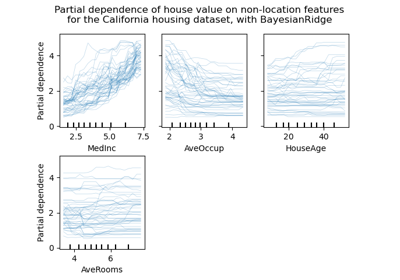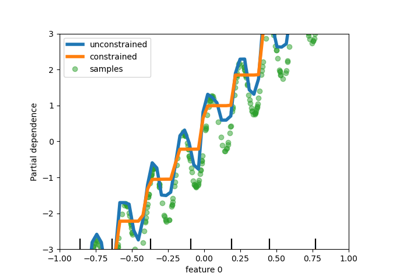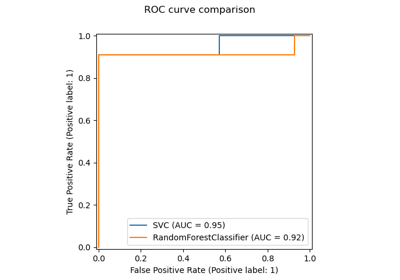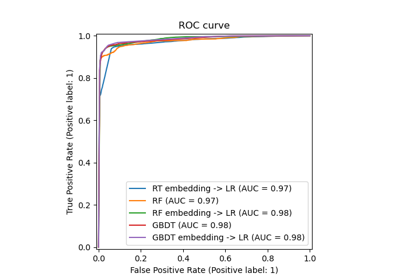# sklearn.ensemble.HistGradientBoostingClassifier¶

class sklearn.ensemble.HistGradientBoostingClassifier(loss='log_loss', *, learning_rate=0.1, max_iter=100, max_leaf_nodes=31, max_depth=None, min_samples_leaf=20, l2_regularization=0.0, max_bins=255, categorical_features=None, monotonic_cst=None, warm_start=False, early_stopping='auto', scoring='loss', validation_fraction=0.1, n_iter_no_change=10, tol=1e-07, verbose=0, random_state=None)[source]

This estimator is much faster than GradientBoostingClassifier for big datasets (n_samples >= 10 000).

This estimator has native support for missing values (NaNs). During training, the tree grower learns at each split point whether samples with missing values should go to the left or right child, based on the potential gain. When predicting, samples with missing values are assigned to the left or right child consequently. If no missing values were encountered for a given feature during training, then samples with missing values are mapped to whichever child has the most samples.

This implementation is inspired by LightGBM.

Read more in the User Guide.

New in version 0.21.

Parameters:
loss{‘log_loss’, ‘auto’, ‘binary_crossentropy’, ‘categorical_crossentropy’}, default=’log_loss’

The loss function to use in the boosting process.

For binary classification problems, ‘log_loss’ is also known as logistic loss, binomial deviance or binary crossentropy. Internally, the model fits one tree per boosting iteration and uses the logistic sigmoid function (expit) as inverse link function to compute the predicted positive class probability.

For multiclass classification problems, ‘log_loss’ is also known as multinomial deviance or categorical crossentropy. Internally, the model fits one tree per boosting iteration and per class and uses the softmax function as inverse link function to compute the predicted probabilities of the classes.

Deprecated since version 1.1: The loss arguments ‘auto’, ‘binary_crossentropy’ and ‘categorical_crossentropy’ were deprecated in v1.1 and will be removed in version 1.3. Use loss='log_loss' which is equivalent.

learning_ratefloat, default=0.1

The learning rate, also known as shrinkage. This is used as a multiplicative factor for the leaves values. Use 1 for no shrinkage.

max_iterint, default=100

The maximum number of iterations of the boosting process, i.e. the maximum number of trees for binary classification. For multiclass classification, n_classes trees per iteration are built.

max_leaf_nodesint or None, default=31

The maximum number of leaves for each tree. Must be strictly greater than 1. If None, there is no maximum limit.

max_depthint or None, default=None

The maximum depth of each tree. The depth of a tree is the number of edges to go from the root to the deepest leaf. Depth isn’t constrained by default.

min_samples_leafint, default=20

The minimum number of samples per leaf. For small datasets with less than a few hundred samples, it is recommended to lower this value since only very shallow trees would be built.

l2_regularizationfloat, default=0

The L2 regularization parameter. Use 0 for no regularization.

max_binsint, default=255

The maximum number of bins to use for non-missing values. Before training, each feature of the input array X is binned into integer-valued bins, which allows for a much faster training stage. Features with a small number of unique values may use less than max_bins bins. In addition to the max_bins bins, one more bin is always reserved for missing values. Must be no larger than 255.

categorical_featuresarray-like of {bool, int} of shape (n_features) or shape (n_categorical_features,), default=None

Indicates the categorical features.

• None : no feature will be considered categorical.

• boolean array-like : boolean mask indicating categorical features.

• integer array-like : integer indices indicating categorical features.

For each categorical feature, there must be at most max_bins unique categories, and each categorical value must be in [0, max_bins -1].

Read more in the User Guide.

New in version 0.24.

monotonic_cstarray-like of int of shape (n_features), default=None

Indicates the monotonic constraint to enforce on each feature. -1, 1 and 0 respectively correspond to a negative constraint, positive constraint and no constraint. Read more in the User Guide.

New in version 0.23.

warm_startbool, default=False

When set to True, reuse the solution of the previous call to fit and add more estimators to the ensemble. For results to be valid, the estimator should be re-trained on the same data only. See the Glossary.

early_stopping‘auto’ or bool, default=’auto’

If ‘auto’, early stopping is enabled if the sample size is larger than 10000. If True, early stopping is enabled, otherwise early stopping is disabled.

New in version 0.23.

scoringstr or callable or None, default=’loss’

Scoring parameter to use for early stopping. It can be a single string (see The scoring parameter: defining model evaluation rules) or a callable (see Defining your scoring strategy from metric functions). If None, the estimator’s default scorer is used. If scoring='loss', early stopping is checked w.r.t the loss value. Only used if early stopping is performed.

validation_fractionint or float or None, default=0.1

Proportion (or absolute size) of training data to set aside as validation data for early stopping. If None, early stopping is done on the training data. Only used if early stopping is performed.

n_iter_no_changeint, default=10

Used to determine when to “early stop”. The fitting process is stopped when none of the last n_iter_no_change scores are better than the n_iter_no_change - 1 -th-to-last one, up to some tolerance. Only used if early stopping is performed.

tolfloat, default=1e-7

The absolute tolerance to use when comparing scores. The higher the tolerance, the more likely we are to early stop: higher tolerance means that it will be harder for subsequent iterations to be considered an improvement upon the reference score.

verboseint, default=0

The verbosity level. If not zero, print some information about the fitting process.

random_stateint, RandomState instance or None, default=None

Pseudo-random number generator to control the subsampling in the binning process, and the train/validation data split if early stopping is enabled. Pass an int for reproducible output across multiple function calls. See Glossary.

Attributes:
classes_array, shape = (n_classes,)

Class labels.

do_early_stopping_bool

Indicates whether early stopping is used during training.

n_iter_int

Number of iterations of the boosting process.

n_trees_per_iteration_int

The number of tree that are built at each iteration. This is equal to 1 for binary classification, and to n_classes for multiclass classification.

train_score_ndarray, shape (n_iter_+1,)

The scores at each iteration on the training data. The first entry is the score of the ensemble before the first iteration. Scores are computed according to the scoring parameter. If scoring is not ‘loss’, scores are computed on a subset of at most 10 000 samples. Empty if no early stopping.

validation_score_ndarray, shape (n_iter_+1,)

The scores at each iteration on the held-out validation data. The first entry is the score of the ensemble before the first iteration. Scores are computed according to the scoring parameter. Empty if no early stopping or if validation_fraction is None.

is_categorical_ndarray, shape (n_features, ) or None

Boolean mask for the categorical features. None if there are no categorical features.

n_features_in_int

Number of features seen during fit.

New in version 0.24.

feature_names_in_ndarray of shape (n_features_in_,)

Names of features seen during fit. Defined only when X has feature names that are all strings.

New in version 1.0.

GradientBoostingClassifier

Exact gradient boosting method that does not scale as good on datasets with a large number of samples.

sklearn.tree.DecisionTreeClassifier

A decision tree classifier.

RandomForestClassifier

A meta-estimator that fits a number of decision tree classifiers on various sub-samples of the dataset and uses averaging to improve the predictive accuracy and control over-fitting.

AdaBoostClassifier

A meta-estimator that begins by fitting a classifier on the original dataset and then fits additional copies of the classifier on the same dataset where the weights of incorrectly classified instances are adjusted such that subsequent classifiers focus more on difficult cases.

Examples

>>> from sklearn.ensemble import HistGradientBoostingClassifier
>>> clf.score(X, y)
1.0


Methods

 Compute the decision function of X. fit(X, y[, sample_weight]) Fit the gradient boosting model. get_params([deep]) Get parameters for this estimator. Predict classes for X. Predict class probabilities for X. score(X, y[, sample_weight]) Return the mean accuracy on the given test data and labels. set_params(**params) Set the parameters of this estimator. Compute decision function of X for each iteration. Predict classes at each iteration. Predict class probabilities at each iteration.
decision_function(X)[source]

Compute the decision function of X.

Parameters:
Xarray-like, shape (n_samples, n_features)

The input samples.

Returns:
decisionndarray, shape (n_samples,) or (n_samples, n_trees_per_iteration)

The raw predicted values (i.e. the sum of the trees leaves) for each sample. n_trees_per_iteration is equal to the number of classes in multiclass classification.

fit(X, y, sample_weight=None)[source]

Parameters:
Xarray-like of shape (n_samples, n_features)

The input samples.

yarray-like of shape (n_samples,)

Target values.

sample_weightarray-like of shape (n_samples,) default=None

Weights of training data.

New in version 0.23.

Returns:
selfobject

Fitted estimator.

get_params(deep=True)[source]

Get parameters for this estimator.

Parameters:
deepbool, default=True

If True, will return the parameters for this estimator and contained subobjects that are estimators.

Returns:
paramsdict

Parameter names mapped to their values.

property n_iter_

Number of iterations of the boosting process.

predict(X)[source]

Predict classes for X.

Parameters:
Xarray-like, shape (n_samples, n_features)

The input samples.

Returns:
yndarray, shape (n_samples,)

The predicted classes.

predict_proba(X)[source]

Predict class probabilities for X.

Parameters:
Xarray-like, shape (n_samples, n_features)

The input samples.

Returns:
pndarray, shape (n_samples, n_classes)

The class probabilities of the input samples.

score(X, y, sample_weight=None)[source]

Return the mean accuracy on the given test data and labels.

In multi-label classification, this is the subset accuracy which is a harsh metric since you require for each sample that each label set be correctly predicted.

Parameters:
Xarray-like of shape (n_samples, n_features)

Test samples.

yarray-like of shape (n_samples,) or (n_samples, n_outputs)

True labels for X.

sample_weightarray-like of shape (n_samples,), default=None

Sample weights.

Returns:
scorefloat

Mean accuracy of self.predict(X) wrt. y.

set_params(**params)[source]

Set the parameters of this estimator.

The method works on simple estimators as well as on nested objects (such as Pipeline). The latter have parameters of the form <component>__<parameter> so that it’s possible to update each component of a nested object.

Parameters:
**paramsdict

Estimator parameters.

Returns:
selfestimator instance

Estimator instance.

staged_decision_function(X)[source]

Compute decision function of X for each iteration.

This method allows monitoring (i.e. determine error on testing set) after each stage.

Parameters:
Xarray-like of shape (n_samples, n_features)

The input samples.

Yields:
decisiongenerator of ndarray of shape (n_samples,) or (n_samples, n_trees_per_iteration)

The decision function of the input samples, which corresponds to the raw values predicted from the trees of the ensemble . The classes corresponds to that in the attribute classes_.

staged_predict(X)[source]

Predict classes at each iteration.

This method allows monitoring (i.e. determine error on testing set) after each stage.

New in version 0.24.

Parameters:
Xarray-like of shape (n_samples, n_features)

The input samples.

Yields:
ygenerator of ndarray of shape (n_samples,)

The predicted classes of the input samples, for each iteration.

staged_predict_proba(X)[source]

Predict class probabilities at each iteration.

This method allows monitoring (i.e. determine error on testing set) after each stage.

Parameters:
Xarray-like of shape (n_samples, n_features)

The input samples.

Yields:
ygenerator of ndarray of shape (n_samples,)

The predicted class probabilities of the input samples, for each iteration.

## Examples using sklearn.ensemble.HistGradientBoostingClassifier¶Release Highlights for scikit-learn 0.24

Release Highlights for scikit-learn 0.24Release Highlights for scikit-learn 0.23

Release Highlights for scikit-learn 0.23Release Highlights for scikit-learn 0.22

Release Highlights for scikit-learn 0.22Feature transformations with ensembles of trees

Feature transformations with ensembles of trees